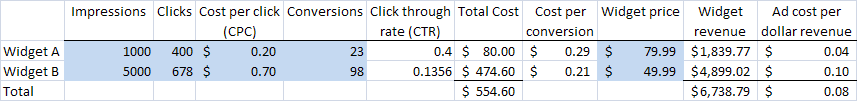Ever wonder if you are spending money wisely on search advertising? I recently had to go through the exercise to understand terminology and analyze if the money we spent on search engine advertising was really worth it. I figured this would be useful to others looking to do similar analysis.

Let’s start with some of the basic terminology used by search engines such as Google, Bing, Yahoo, etc. These metrics are provided to you by the search engines based on your keyword.

Impressions: The number of times a target customer searching for a keyword sees your advertised link. This usually shows up as a sponsored link on top of a Google search page in a green background.

Clicks: The number of times the target customer actually clicks on the advertized link.

Calculated metrics:

Using the numbers above we can easily create the metrics below that can be used to assess the efficiency and economics of your keywords.

Click through rate (CTR): The CTR is a measure of how relevant your advertisement is to the customer’s search phrase. This is calculated as the Clicks divided by the number of impressions.

Total Cost: This is the total dollar amount spent on search engine advertising. This is calculated by multiplying the CPC by the number of clicks.

Cost per conversion: This metric gives the average dollar amount you spent for making one sale. This number is calculated by dividing the total cost by the number of conversions.

Cost per revenue: This metric gives you the average cents you spent to earn one dollar. This number is simply calculated by dividing the total sales you had to customers (who clicked on the advertising link and bought your product or service) by the total cost.

Example:

The table below shows examples of these calculations for an imaginary business selling two widgets as well as the total costs, revenue and average advertising costs per dollar revenue. The numbers highlighted in blue can be obtained from the search engine. In this example the business spends 8 cents of every dollar earned on search engine advertising.